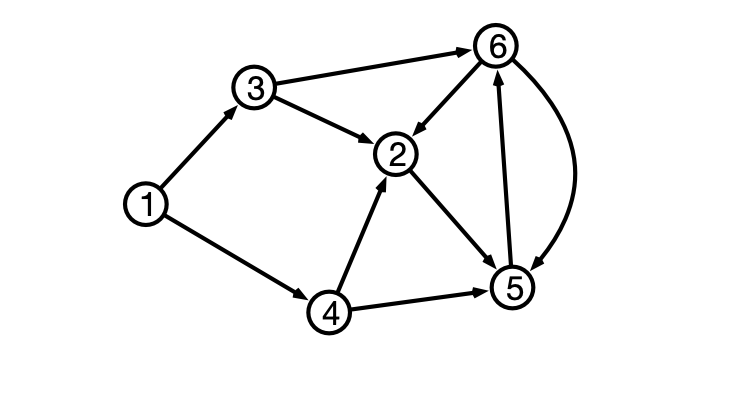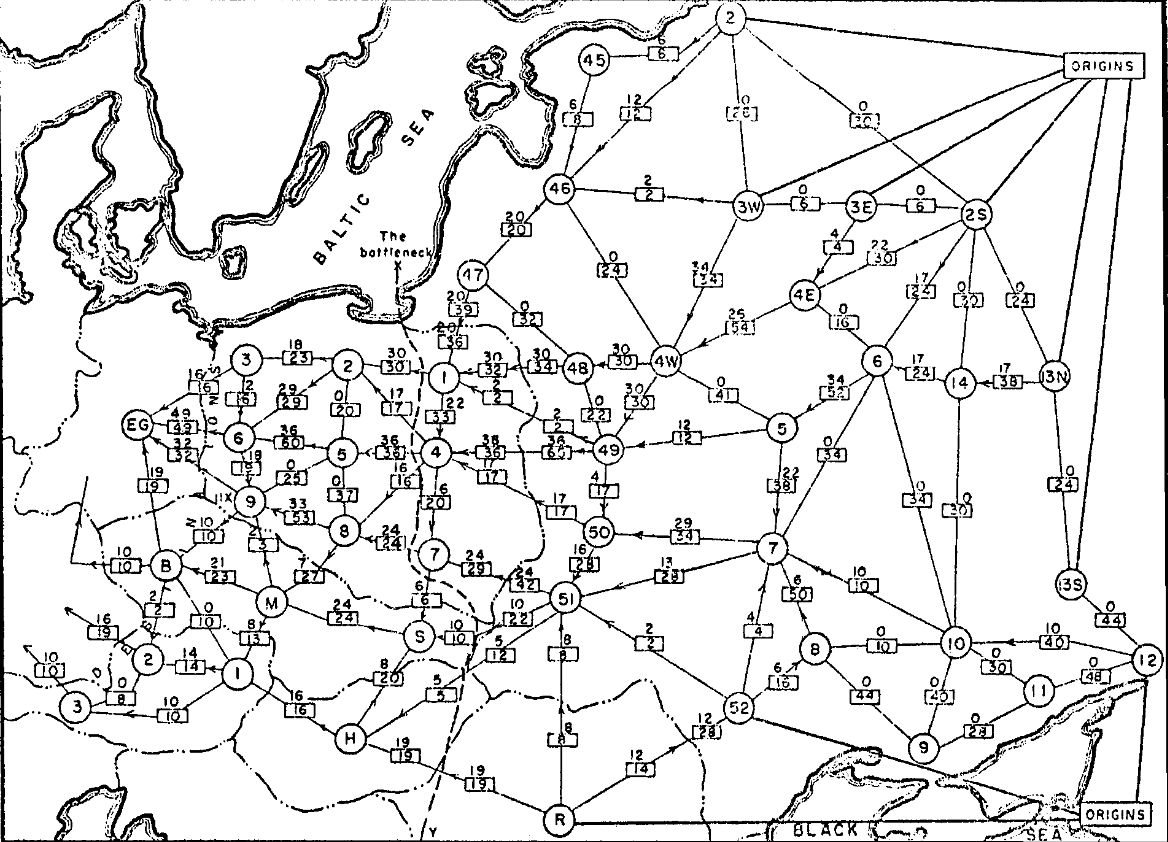# Lecture 27: Shortest Paths, Revisited

$\def\opt{ {\mathrm{opt}} }$

## Announcements

1. HW 05 Due this Friday
2. Midterm 2 Wednesday 11/16
• in class
• focus on material since last midterm up to this Friday
• divide and conquer
• greedy
• dynamic programming
• network flow
• mixture of computational and algorithm design questions
• details forthcoming

## Directed Graphs and Paths## Representing Directed Graphs

• $v$’s neighbors are outgoing neighbors## Single Source Shortest Paths

Input:

• (Directed) graph $G = (V, E)$, edge weights $w$
• Starting vertex $u$

Output:

• $d(v) =$ distance from $u$ to $v$ for every vertex $v$
• distance is length of shortest directed path from $u$ to $v$
• length is sum of edge weights of path

## Previous Algorithms

• solves SSSP when all edge weights are $1$
2. Dijkstra’s Algorithm
• solves SSSP when all edge weights are $\geq 0$

Question. What if edge weights can be negative?

## Example 1

Question 1. Why can Dijkstra fail when edge weights can be negative?

## Example 2

Question 2. Why might shortest paths not exist when edge weights can be negative?

## Assumption

Assume. $G$ does not contain any negative weight cycles.

Claim. $G$ a graph with $n$ vertices, $u, v$ vertices in $G$. If $G$ does not contain negative weight cycles, then the shortest (weighted) path from $u$ to $v$ contains at most $n-1$ edges.

Why?

## Shortest Paths Again

Suppose shortest path from $u$ to $x$ contains $j$ hops.

• $v$ is $x$’s “parent” along path
• $d(u, x) = d(u, v) + w(v, x)$
• shortest path from $u$ to $v$ has $j-1$ hops

## Dynamic Programming Approach

Idea. For each vertex $v$ and each $j = 1, 2, \ldots, n-1$ compute $d_j(u, v) =$ length of shortest path from $u$ to $v$ with at most $j$ hops.

• Note $d(u, v) = d_{n-1}(u, v)$.

## Questions

Question 1. How to initialize $d_0(u, v)$?

Question 2. Given $d_j(u, v)$ for all v, how to find $d_{j+1}(u, v)$?

## Bellman-Ford Algorithm

  Bellman-Ford(V, E, w, u)
d <- 2d array [0..n-1, 1..n]
for v = 1 to n do d[0, v] <- infinity
d[0, u] <- 0
for j = 1 to n-1 do
for each vertex v in V set d[j, v] <- d[j-1,v]
for each vertex v in V
for each neighbor x of v
d[j, x] <- Min(d[j, x], d[j-1, v] + w[v, x])
return d[n-1]


Running time?

## Correctness

Claim. For all $j = 0, 1, \ldots, n-1$ and for all vertices $v$, $d[j, v]$ stores length of shortest path from $u$ to $v$ with $j$ or fewer hops. I.e., $d[j, v] = d_j(v)$

Proof. Induction on $j$.

Base case, $j = 0$.

## Inductive Step, $j-1 \implies j$

• suppose $d[j-1, v] = d_{j-1}(v)$ for all $v$
• consider shortest path $P$ of $j$ hops from $u$ to $v$
• let $x$ be penultimate vertex in $P$
• then $d_j(v) = d_{j-1}(x) + w(x, v)$
• by inductive hypothesis, $d_{j-1}(x) = d[j-1,x]$
• therefore in iteration $j$, get $d[j, v] \leq d[j-1,x] + w(x, v) = d_{j-1}(x) + w(x, v) = d_j(v)$
• also have $d[j, v] \geq d_j(v)$ (why?)
• so $d[j, v] = d_j(v)$

## Conclusion

If $G$ has no negative weight cycles, then Bellman-Ford solves single source shortest paths in $O(m n)$ time.

## Dijkstra vs Bellman-Ford?

Running times:

• Dijkstra: $O(m \log n)$
• Bellman-Ford: $O(m n)$

Why pick Bellman-Ford over Dijkstra?

• Why might Bellman-Ford be preferable even if graph has no negative weight edges?

## Bellman-Ford Again

  Bellman-Ford(V, E, w, u)
d <- 2d array [0..n-1, 1..n]
for v = 1 to n do d[0, v] <- infinity
d[0, u] <- 0
for j = 1 to n-1 do
for each vertex v in V set d[j, v] <- d[j-1,v]
for each vertex v in V
for each neighbor x of v
d[j, x] <- Min(d[j, x], d[j-1, v] + w[v, x])
return d[n-1]


# Next Time: Cold War

## Rail Network of Eastern Europe## Networks to Graphs

Modeling the network:

• nodes represent railway junctions
• edges represent rail lines
• weights represent capacities of lines
• capacity indicates tonnage that can cross line per unit time
• proportional to cost of disrupting movement along line

Question 1. How much material can the USSR transport to Western Europe per unit time?

Question 2. What is the cheapest way to disrupt flow of material?

## Network Flow

A new interpretation of directed graphs:

• network of (directional) pipes
• weights are capacities
• how much fluid can flow through piper per time
• designated source node $s$
• all edges directed away from $s$
• designated sink or destination node $t$
• all edges directed towards $t$

Question. How much fluid be routed from $s$ to $t$ per unit time?